xSpinorEncyclopedia
In mathematics
Mathematics
Mathematics is the study of quantity, space, structure, and change. Mathematicians seek out patterns and formulate new conjectures. Mathematicians resolve the truth or falsity of conjectures by mathematical proofs, which are arguments sufficient to convince other mathematicians of their validity...

and physics
Physics
Physics is a natural science that involves the study of matter and its motion through spacetime, along with related concepts such as energy and force. More broadly, it is the general analysis of nature, conducted in order to understand how the universe behaves.Physics is one of the oldest academic...

, in particular in the theory of the orthogonal group
Orthogonal group
In mathematics, the orthogonal group of degree n over a field F is the group of n × n orthogonal matrices with entries from F, with the group operation of matrix multiplication...

s (such as the rotation
Rotation group
In mechanics and geometry, the rotation group is the group of all rotations about the origin of three-dimensional Euclidean space R3 under the operation of composition. By definition, a rotation about the origin is a linear transformation that preserves length of vectors and preserves orientation ...

or the Lorentz group
Lorentz group
In physics , the Lorentz group is the group of all Lorentz transformations of Minkowski spacetime, the classical setting for all physical phenomena...

s), spinors are elements of a complex vector space
Vector space
A vector space is a mathematical structure formed by a collection of vectors: objects that may be added together and multiplied by numbers, called scalars in this context. Scalars are often taken to be real numbers, but one may also consider vector spaces with scalar multiplication by complex...

introduced to expand the notion of spatial vector. Unlike tensors, the space of spinors cannot be built up in a unique and natural way from spatial vectors. However, spinors transform well under the infinitesimal orthogonal transformations (like infinitesimal rotation
Rotation
A rotation is a circular movement of an object around a center of rotation. A three-dimensional object rotates always around an imaginary line called a rotation axis. If the axis is within the body, and passes through its center of mass the body is said to rotate upon itself, or spin. A rotation...

s or infinitesimal Lorentz transformation
Lorentz transformation
In physics, the Lorentz transformation or Lorentz-Fitzgerald transformation describes how, according to the theory of special relativity, two observers' varying measurements of space and time can be converted into each other's frames of reference. It is named after the Dutch physicist Hendrik...

s). Under the full orthogonal group, however, they do not quite transform well, but only "up to a sign". This means that a 360 degree
Turn (geometry)
A turn is an angle equal to a 360° or 2 radians or \tau radians. A turn is also referred to as a revolution or complete rotation or full circle or cycle or rev or rot....

rotation transforms a spinor into its negative, and so it takes a rotation of 720 degrees for a spinor to be transformed into itself. Specifically, spinors are objects associated to a vector space with a quadratic form
In mathematics, a quadratic form is a homogeneous polynomial of degree two in a number of variables. For example,4x^2 + 2xy - 3y^2\,\!is a quadratic form in the variables x and y....

(like Euclidean space
Euclidean space
In mathematics, Euclidean space is the Euclidean plane and three-dimensional space of Euclidean geometry, as well as the generalizations of these notions to higher dimensions...

with the standard metric or Minkowski space
Minkowski space
In physics and mathematics, Minkowski space or Minkowski spacetime is the mathematical setting in which Einstein's theory of special relativity is most conveniently formulated...

with the Lorentz metric), and are realized as elements of representation spaces of Clifford algebras. For a given quadratic form, several different spaces of spinors with extra properties may exist.

Spinors in general were discovered by Élie Cartan
Élie Cartan
Élie Joseph Cartan was an influential French mathematician, who did fundamental work in the theory of Lie groups and their geometric applications...

in 1913. Later, spinors were adopted by quantum mechanics
Quantum mechanics
Quantum mechanics, also known as quantum physics or quantum theory, is a branch of physics providing a mathematical description of much of the dual particle-like and wave-like behavior and interactions of energy and matter. It departs from classical mechanics primarily at the atomic and subatomic...

in order to study the properties of the intrinsic angular momentum of the electron
Electron
The electron is a subatomic particle with a negative elementary electric charge. It has no known components or substructure; in other words, it is generally thought to be an elementary particle. An electron has a mass that is approximately 1/1836 that of the proton...

and other fermion
Fermion
In particle physics, a fermion is any particle which obeys the Fermi–Dirac statistics . Fermions contrast with bosons which obey Bose–Einstein statistics....

s. Today spinors enjoy a wide range of physics applications. Classically, spinors in three dimensions
Spinors in three dimensions
In mathematics, the spinor concept as specialised to three dimensions can be treated by means of the traditional notions of dot product and cross product. This is part of the detailed algebraic discussion of the rotation group SO.-Formulation:...

are used to describe the spin of the non-relativistic electron and other spin-½
Spin-½
In quantum mechanics, spin is an intrinsic property of all elementary particles. Fermions, the particles that constitute ordinary matter, have half-integer spin. Spin-½ particles constitute an important subset of such fermions. All known elementary fermions have a spin of ½.- Overview :Particles...

particles. Via the Dirac equation
Dirac equation
The Dirac equation is a relativistic quantum mechanical wave equation formulated by British physicist Paul Dirac in 1928. It provided a description of elementary spin-½ particles, such as electrons, consistent with both the principles of quantum mechanics and the theory of special relativity, and...

, Dirac spinors are required in the mathematical description of the quantum state of the relativistic
Theory of relativity
The theory of relativity, or simply relativity, encompasses two theories of Albert Einstein: special relativity and general relativity. However, the word relativity is sometimes used in reference to Galilean invariance....

electron. In quantum field theory
Quantum field theory
Quantum field theory provides a theoretical framework for constructing quantum mechanical models of systems classically parametrized by an infinite number of dynamical degrees of freedom, that is, fields and many-body systems. It is the natural and quantitative language of particle physics and...

, spinors describe the state of relativistic many-particle systems. In mathematics, particularly in differential geometry and global analysis, spinors have since found broad applications to algebraic
Algebraic topology
Algebraic topology is a branch of mathematics which uses tools from abstract algebra to study topological spaces. The basic goal is to find algebraic invariants that classify topological spaces up to homeomorphism, though usually most classify up to homotopy equivalence.Although algebraic topology...

and differential topology
Differential topology
In mathematics, differential topology is the field dealing with differentiable functions on differentiable manifolds. It is closely related to differential geometry and together they make up the geometric theory of differentiable manifolds.- Description :...

, symplectic geometry, gauge theory
Gauge theory
In physics, gauge invariance is the property of a field theory in which different configurations of the underlying fundamental but unobservable fields result in identical observable quantities. A theory with such a property is called a gauge theory...

, complex algebraic geometry, index theory, and special holonomy
Holonomy
In differential geometry, the holonomy of a connection on a smooth manifold is a general geometrical consequence of the curvature of the connection measuring the extent to which parallel transport around closed loops fails to preserve the geometrical data being transported. For flat connections,...

.

## Overview

In the classical geometry of space, a vector exhibits a certain behavior when it is acted upon by a rotation or reflected in a hyperplane. However, in a certain sense rotations and reflections contain finer geometrical information than can be expressed in terms of their actions on vectors. Spinors are objects constructed in order to encompass more fully this geometry. (See orientation entanglement
Orientation entanglement
In mathematics and physics, the notion of orientation entanglement is sometimes used to develop intuition relating to the geometry of spinors or alternatively as a concrete realization of the failure of the special orthogonal groups to be simply connected....

.)

There are essentially two frameworks for viewing the notion of a spinor.

One is representation theoretic
Representation theory
Representation theory is a branch of mathematics that studies abstract algebraic structures by representing their elements as linear transformations of vector spaces, and studiesmodules over these abstract algebraic structures...

. In this point of view, one knows a priori that there are some representations of the Lie algebra
Lie algebra
In mathematics, a Lie algebra is an algebraic structure whose main use is in studying geometric objects such as Lie groups and differentiable manifolds. Lie algebras were introduced to study the concept of infinitesimal transformations. The term "Lie algebra" was introduced by Hermann Weyl in the...

of the orthogonal group
Orthogonal group
In mathematics, the orthogonal group of degree n over a field F is the group of n × n orthogonal matrices with entries from F, with the group operation of matrix multiplication...

which cannot be formed by the usual tensor constructions. These missing representations are then labeled the spin representations, and their constituents spinors. In this view, a spinor must belong to a representation
Group representation
In the mathematical field of representation theory, group representations describe abstract groups in terms of linear transformations of vector spaces; in particular, they can be used to represent group elements as matrices so that the group operation can be represented by matrix multiplication...

of the double cover of the rotation group
Rotation group
In mechanics and geometry, the rotation group is the group of all rotations about the origin of three-dimensional Euclidean space R3 under the operation of composition. By definition, a rotation about the origin is a linear transformation that preserves length of vectors and preserves orientation ...

, or more generally of the generalized special orthogonal group  on spaces with metric signature
Metric signature
The signature of a metric tensor is the number of positive and negative eigenvalues of the metric. That is, the corresponding real symmetric matrix is diagonalised, and the diagonal entries of each sign counted...

. These double-covers are Lie groups, called the spin groups . All the properties of spinors, and their applications and derived objects, are manifested first in the spin group.

The other point of view is geometrical. One can explicitly construct the spinors, and then examine how they behave under the action of the relevant Lie groups. This latter approach has the advantage of providing a concrete and elementary description of what a spinor is. However, such a description becomes unwieldy when complicated properties of spinors, such as Fierz identities
Fierz identity
In theoretical physics, a Fierz identity is an identity that allows one to rewrite bilinears of the product of two spinors as a linear combination of products of the bilinears of the individual spinors. It is named after Swiss physicist Markus Fierz....

, are needed.

### Clifford algebras

The language of Clifford algebra
Clifford algebra
In mathematics, Clifford algebras are a type of associative algebra. As K-algebras, they generalize the real numbers, complex numbers, quaternions and several other hypercomplex number systems. The theory of Clifford algebras is intimately connected with the theory of quadratic forms and orthogonal...

s (also called geometric algebra
Geometric algebra
Geometric algebra , together with the associated Geometric calculus, provides a comprehensive alternative approach to the algebraic representation of classical, computational and relativistic geometry. GA now finds application in all of physics, in graphics and in robotics...

s) provides a complete picture of the spin representations of all the spin groups, and the various relationships between those representations, via the classification of Clifford algebras
Classification of Clifford algebras
In abstract algebra, in particular in the theory of nondegenerate quadratic forms on vector spaces, the structures of finite-dimensional real and complex Clifford algebras have been completely classified...

. It largely removes the need for ad hoc constructions.

In detail, if V is a finite-dimensional complex vector space with nondegenerate bilinear form g, the Clifford algebra is the algebra generated by V along with the anticommutation relation . It is an abstract version of the algebra generated by the gamma or Pauli matrices
Pauli matrices
The Pauli matrices are a set of three 2 × 2 complex matrices which are Hermitian and unitary. Usually indicated by the Greek letter "sigma" , they are occasionally denoted with a "tau" when used in connection with isospin symmetries...

. The Clifford algebra Cℓn(C) is algebraically isomorphic to the algebra of complex matrices, if is even; or the algebra of two copies of the matrices, if is odd. It therefore has a unique irreducible representation (also called simple Clifford module), commonly denoted by Δ, whose dimension is 2k. The Lie algebra is embedded as a Lie subalgebra in equipped with the Clifford algebra commutator
Commutator
In mathematics, the commutator gives an indication of the extent to which a certain binary operation fails to be commutative. There are different definitions used in group theory and ring theory.-Group theory:...

as Lie bracket. Therefore, the space Δ is also a Lie algebra representation of called a spin representation. If n is odd, this representation is irreducible. If n is even, it splits again into two irreducible representations called the half-spin representations.

Irreducible representations over the reals in the case when V is a real vector space are much more intricate, and the reader is referred to the Clifford algebra article for more details.

### Terminology in physics

The most typical type of spinor, the Dirac spinor, is an element of the fundamental representation of the complexified Clifford algebra
Clifford algebra
In mathematics, Clifford algebras are a type of associative algebra. As K-algebras, they generalize the real numbers, complex numbers, quaternions and several other hypercomplex number systems. The theory of Clifford algebras is intimately connected with the theory of quadratic forms and orthogonal...

, into which the spin group Spin(p, q) may be embedded. On a 2k- or 2k+1-dimensional space a Dirac spinor may be represented as a vector of 2k complex number
Complex number
A complex number is a number consisting of a real part and an imaginary part. Complex numbers extend the idea of the one-dimensional number line to the two-dimensional complex plane by using the number line for the real part and adding a vertical axis to plot the imaginary part...

s. (See Special unitary group
Special unitary group
The special unitary group of degree n, denoted SU, is the group of n×n unitary matrices with determinant 1. The group operation is that of matrix multiplication...

.) In even dimensions, this representation is reducible when taken as a representation of and may be decomposed into two: the left-handed and right-handed Weyl spinor representations. In addition, sometimes the non-complexified version of has a smaller real representation, the Majorana spinor representation. If this happens in an even dimension, the Majorana spinor representation will sometimes decompose into two Majorana–Weyl spinor representations.

Of all these, only the Dirac representation exists in all dimensions. Dirac and Weyl spinors are complex representations while Majorana spinors are real representations.

### Spinors in representation theory

One major mathematical application of the construction of spinors is to make possible the explicit construction of linear representations of the Lie algebra
Lie algebra
In mathematics, a Lie algebra is an algebraic structure whose main use is in studying geometric objects such as Lie groups and differentiable manifolds. Lie algebras were introduced to study the concept of infinitesimal transformations. The term "Lie algebra" was introduced by Hermann Weyl in the...

s of the special orthogonal groups, and consequently spinor representations of the groups themselves. At a more profound level, spinors have been found to be at the heart of approaches to the index theorem, and to provide constructions in particular for discrete series representations of semisimple groups.

The spin representations of the special orthogonal Lie algebras are distinguished from the tensor
Tensor
Tensors are geometric objects that describe linear relations between vectors, scalars, and other tensors. Elementary examples include the dot product, the cross product, and linear maps. Vectors and scalars themselves are also tensors. A tensor can be represented as a multi-dimensional array of...

representations given by Weyl's construction
Young symmetrizer
In mathematics, a Young symmetrizer is an element of the group algebra of the symmetric group, constructed in such a way that the image of the element corresponds to an irreducible representation of the symmetric group over the complex numbers. A similar construction works over any field, and the...

by the weights
Weight (representation theory)
In the mathematical field of representation theory, a weight of an algebra A over a field F is an algebra homomorphism from A to F – a linear functional – or equivalently, a one dimensional representation of A over F. It is the algebra analogue of a multiplicative character of a group...

. Whereas the weights of the tensor representations are integer linear combinations of the roots of the Lie algebra, those of the spin representations are half-integer linear combinations thereof. Explicit details can be found in the spin representation article.

### History

The most general mathematical form of spinors was discovered by Élie Cartan
Élie Cartan
Élie Joseph Cartan was an influential French mathematician, who did fundamental work in the theory of Lie groups and their geometric applications...

in 1913. The word "spinor" was coined by Paul Ehrenfest
Paul Ehrenfest
Paul Ehrenfest was an Austrian and Dutch physicist, who made major contributions to the field of statistical mechanics and its relations with quantum mechanics, including the theory of phase transition and the Ehrenfest theorem.- Biography :Paul Ehrenfest was born and grew up in Vienna in a Jewish...

in his work on quantum physics.

Spinors were first applied to mathematical physics
Mathematical physics
Mathematical physics refers to development of mathematical methods for application to problems in physics. The Journal of Mathematical Physics defines this area as: "the application of mathematics to problems in physics and the development of mathematical methods suitable for such applications and...

by Wolfgang Pauli
Wolfgang Pauli
Wolfgang Ernst Pauli was an Austrian theoretical physicist and one of the pioneers of quantum physics. In 1945, after being nominated by Albert Einstein, he received the Nobel Prize in Physics for his "decisive contribution through his discovery of a new law of Nature, the exclusion principle or...

in 1927, when he introduced spin matrices
Pauli matrices
The Pauli matrices are a set of three 2 × 2 complex matrices which are Hermitian and unitary. Usually indicated by the Greek letter "sigma" , they are occasionally denoted with a "tau" when used in connection with isospin symmetries...

. The following year, Paul Dirac
Paul Dirac
Paul Adrien Maurice Dirac, OM, FRS was an English theoretical physicist who made fundamental contributions to the early development of both quantum mechanics and quantum electrodynamics...

discovered the fully relativistic
Special relativity
Special relativity is the physical theory of measurement in an inertial frame of reference proposed in 1905 by Albert Einstein in the paper "On the Electrodynamics of Moving Bodies".It generalizes Galileo's...

theory of electron
Electron
The electron is a subatomic particle with a negative elementary electric charge. It has no known components or substructure; in other words, it is generally thought to be an elementary particle. An electron has a mass that is approximately 1/1836 that of the proton...

spin
Spin (physics)
In quantum mechanics and particle physics, spin is a fundamental characteristic property of elementary particles, composite particles , and atomic nuclei.It is worth noting that the intrinsic property of subatomic particles called spin and discussed in this article, is related in some small ways,...

by showing the connection between spinors and the Lorentz group
Lorentz group
In physics , the Lorentz group is the group of all Lorentz transformations of Minkowski spacetime, the classical setting for all physical phenomena...

. By the 1930s, Dirac, Piet Hein
Piet Hein (Denmark)
Piet Hein was a Danish scientist, mathematician, inventor, designer, author, and poet, often writing under the Old Norse pseudonym "Kumbel" meaning "tombstone"...

and others at the Niels Bohr Institute
Niels Bohr Institute
The Niels Bohr Institute is a research institute of the University of Copenhagen. The research of the institute spans astronomy, geophysics, nanotechnology, particle physics, quantum mechanics and biophysics....

created games such as Tangloids
Tangloids
Tangloids is a mathematical game for two players created by Piet Hein to model the calculus of spinors.Two flat blocks of wood each pierced with three tiny holes are joined with three parallel strings. Each player holds one of the blocks of wood. The first player holds one block of wood still,...

to teach and model the calculus of spinors.

## Examples

Some simple examples of spinors in low dimensions arise from considering the even-graded subalgebras of the Clifford algebra . This is an algebra built up from an orthonormal basis of mutually orthogonal vectors under addition and multiplication, p of which have norm +1 and q of which have norm −1, with the product rule for the basis vectors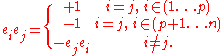### Two dimensions

The Clifford algebra Cℓ2,0(R) is built up from a basis of one unit scalar, 1, two orthogonal unit vectors, σ1 and σ2, and one unit pseudoscalar
Pseudoscalar
In physics, a pseudoscalar is a quantity that behaves like a scalar, except that it changes sign under a parity inversion such as improper rotations while a true scalar does not.The prototypical example of a pseudoscalar is the scalar triple product...

. From the definitions above, it is evident that , and .

The even subalgebra Cℓ02,0(R), spanned by even-graded basis elements of Cℓ2,0(R), determines the space of spinors via its representations. It is made up of real linear combinations of 1 and σ1σ2. As a real algebra, Cℓ02,0(R) is isomorphic to field of complex numbers C. As a result, it admits a conjugation operation (analogous to complex conjugation
Complex conjugate
In mathematics, complex conjugates are a pair of complex numbers, both having the same real part, but with imaginary parts of equal magnitude and opposite signs...

), sometimes called the reverse of a Clifford element, defined by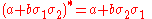.
which, by the Clifford relations, can be written.

The action of an even Clifford element on vectors, regarded as 1-graded elements of Cℓ2,0, is determined by mapping a general vector to the vector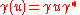,
where γ* is the conjugate of γ, and the product is Clifford multiplication. In this situation, a spinor is an ordinary complex number. The action of γ on a spinor φ is given by ordinary complex multiplication: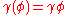.

An important feature of this definition is the distinction between ordinary vectors and spinors, manifested in how the even-graded elements act on each of them in different ways. In general, a quick check of the Clifford relations reveals that even-graded elements conjugate-commute with ordinary vectors:.
On the other hand, comparing with the action on spinors , γ on ordinary vectors acts as the square of its action on spinors.

Consider, for example, the implication this has for plane rotations. Rotating a vector through an angle of θ corresponds to , so that the corresponding action on spinors is via . In general, because of logarithmic branching, it is impossible to choose a sign in a consistent way. Thus the representation of plane-rotations on spinors is two-valued.

In applications of spinors in two dimensions, it is common to exploit the fact that the algebra of even-graded elements (which is just the ring of complex numbers) is identical to the space of spinors. So, by abuse of language
Abuse of notation
In mathematics, abuse of notation occurs when an author uses a mathematical notation in a way that is not formally correct but that seems likely to simplify the exposition or suggest the correct intuition . Abuse of notation should be contrasted with misuse of notation, which should be avoided...

, the two are often conflated. One may then talk about "the action of a spinor on a vector." In a general setting, such statements are meaningless. But in dimensions 2 and 3 (as applied, for example, to computer graphics
Computer graphics
Computer graphics are graphics created using computers and, more generally, the representation and manipulation of image data by a computer with help from specialized software and hardware....

) they make sense.

Examples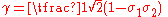corresponds to a vector rotation of 90° from σ1 around towards σ2, which can be checked by confirming that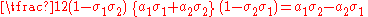It corresponds to a spinor rotation of only 45°, however:• Similarly the even-graded element γ = −σ1σ2 corresponds to a vector rotation of 180°:but a spinor rotation of only 90°: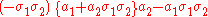• Continuing on further, the even-graded element γ = −1 corresponds to a vector rotation of 360°: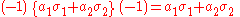but a spinor rotation of 180°.

### Three dimensions

Main articles Spinors in three dimensions
Spinors in three dimensions
In mathematics, the spinor concept as specialised to three dimensions can be treated by means of the traditional notions of dot product and cross product. This is part of the detailed algebraic discussion of the rotation group SO.-Formulation:...

, Quaternions and spatial rotation
Quaternions and spatial rotation
Unit quaternions provide a convenient mathematical notation for representing orientations and rotations of objects in three dimensions. Compared to Euler angles they are simpler to compose and avoid the problem of gimbal lock. Compared to rotation matrices they are more numerically stable and may...

The Clifford algebra Cℓ3,0(R) is built up from a basis of one unit scalar, 1, three orthogonal unit vectors, σ1, σ2 and σ3, the three unit bivectors σ1σ2, σ2σ3, σ3σ1 and the pseudoscalar
Pseudoscalar
In physics, a pseudoscalar is a quantity that behaves like a scalar, except that it changes sign under a parity inversion such as improper rotations while a true scalar does not.The prototypical example of a pseudoscalar is the scalar triple product...

i = σ1σ2σ3. It is straightforward to show that (σ1)2 = (σ2)2 = (σ3)2 = 1, and
1σ2)2 = (σ2σ3)2 = (σ3σ1)2 = (σ1σ2σ3)2 = −1.

The sub-algebra of even-graded elements is made up of scalar dilations,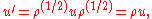and vector rotations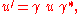where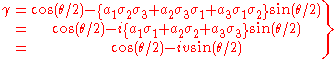(1)
corresponds to a vector rotation through an angle θ about an axis defined by a unit vector v = a1σ1 + a2σ2 + a3σ3

As a special case, it is easy to see that if v = σ3 this reproduces the σ1σ2 rotation considered in the previous section; and that such rotation leaves the coefficients of vectors in the σ3 direction invariant, since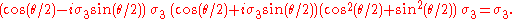The bivectors σ2σ3, σ3σ1 and σ1σ2 are in fact Hamilton's
William Rowan Hamilton
Sir William Rowan Hamilton was an Irish physicist, astronomer, and mathematician, who made important contributions to classical mechanics, optics, and algebra. His studies of mechanical and optical systems led him to discover new mathematical concepts and techniques...

quaternion
Quaternion
In mathematics, the quaternions are a number system that extends the complex numbers. They were first described by Irish mathematician Sir William Rowan Hamilton in 1843 and applied to mechanics in three-dimensional space...

s i, j and k, discovered in 1843:With the identification of the even-graded elements with the algebra H of quaternions, as in the case of two-dimensions the only representation of the algebra of even-graded elements is on itself. Thus the (real) spinors in three-dimensions are quaternions, and the action of an even-graded element on a spinor is given by ordinary quaternionic multiplication.

Note that the expression (1) for a vector rotation through an angle θ, the angle appearing in γ was halved. Thus the spinor rotation γ(ψ) = γψ (ordinary quaternionic multiplication) will rotate the spinor ψ through an angle one-half the measure of the angle of the corresponding vector rotation. Once again, the problem of lifting a vector rotation to a spinor rotation is two-valued: the expression (1) with (180° + θ/2) in place of θ/2 will produce the same vector rotation, but the negative of the spinor rotation.

The spinor/quaternion representation of rotations in 3D is becoming increasingly prevalent in computer geometry and other applications, because of the notable brevity of the corresponding spin matrix, and the simplicity with which they can be multiplied together to calculate the combined effect of successive rotations about different axes.

## Explicit constructions

A space of spinors can be constructed explicitly with concrete and abstract constructions. The
equivalence of these constructions are a consequence of the uniqueness of the spinor representation of the complex Clifford algebra. For a complete example in dimension 3, see spinors in three dimensions
Spinors in three dimensions
In mathematics, the spinor concept as specialised to three dimensions can be treated by means of the traditional notions of dot product and cross product. This is part of the detailed algebraic discussion of the rotation group SO.-Formulation:...

.

### Component spinors

Given a vector space V and a quadratic form g an explicit matrix representation of the Clifford algebra can be defined as follows. Choose an orthonormal basis for V i.e. where and for . Let . Fix a set of matrices such that (i.e. fix a convention for the gamma matrices). Then the assignment extends uniquely to an algebra homomorphism by sending the monomial in the Clifford algebra to the product of matrices and extending linearly. The space on which the gamma matrices act is a now a space of spinors. One needs to construct such matrices explicitly, however. In dimension 3, defining the gamma matrices to be the Pauli sigma matrices
Pauli matrices
The Pauli matrices are a set of three 2 × 2 complex matrices which are Hermitian and unitary. Usually indicated by the Greek letter "sigma" , they are occasionally denoted with a "tau" when used in connection with isospin symmetries...

gives rise to the familiar two component spinors used in non relativistic quantum mechanics
Quantum mechanics
Quantum mechanics, also known as quantum physics or quantum theory, is a branch of physics providing a mathematical description of much of the dual particle-like and wave-like behavior and interactions of energy and matter. It departs from classical mechanics primarily at the atomic and subatomic...

. Likewise using the 4×4 Dirac gamma matrices gives rise to the 4 component Dirac spinors used in 3+1 dimensional relativistic quantum field theory
Quantum field theory
Quantum field theory provides a theoretical framework for constructing quantum mechanical models of systems classically parametrized by an infinite number of dynamical degrees of freedom, that is, fields and many-body systems. It is the natural and quantitative language of particle physics and...

. In general, in order to define gamma matrices of the required kind, one can use the Weyl-Brauer matrices
Weyl-Brauer matrices
In mathematics, particularly in the theory of spinors, the Weyl–Brauer matrices are an explicit realization of a Clifford algebra as a matrix algebra. They generalize in n dimensions as the Pauli matrices...

.

In this construction the representation of the Clifford algebra Cℓ(V, g), the Lie algebra so(V, g), and the Spin group Spin(V, g), all depend on the choice of the orthonormal basis and the choice of the gamma matrices. This can cause confusion
over conventions, but invariants like traces are independent of choices. In particular, all physically observable quantities must be independent of such choices. In this construction a spinor can be represented as a vector of 2k complex numbers and is denoted with spinor indices (usually α, β, γ). In the physics literature, abstract spinor indices are often used to denote spinors even when an abstract spinor construction is used.

### Abstract spinors

There are at least two different, but essentially equivalent, ways to define spinors abstractly. One approach seeks to identify the minimal ideals for the left action of Cℓ(V, g) on itself. These are subspaces of the Clifford algebra of the form Cℓ(V, g)ω, admitting the evident action of Cℓ(V, g) by left-multiplication: c : xω → cxω. There are two variations on this theme: one can either find a primitive element ω which is a nilpotent
Nilpotent
In mathematics, an element x of a ring R is called nilpotent if there exists some positive integer n such that xn = 0....

element of the Clifford algebra, or one which is an idempotent. The construction via nilpotent elements is more fundamental in the sense that an idempotent may then be produced from it. In this way, the spinor representations are identified with certain subspaces of the Clifford algebra itself. The second approach is to construct a vector space using a distinguished subspace of V, and then specify the action of the Clifford algebra externally to that vector space.

In either approach, the fundamental notion is that of an isotropic subspace W. Each construction depends on an initial freedom in choosing this subspace. In physical terms, this corresponds to the fact that there is no measurement protocol which can specify a basis of the spin space, even if a preferred basis of V is given.

As above, we let (V, g) be an n-dimensional complex vector space equipped with a nondegenerate bilinear form. If V is a real vector space, then we replace V by its complexification
Complexification
In mathematics, the complexification of a real vector space V is a vector space VC over the complex number field obtained by formally extending scalar multiplication to include multiplication by complex numbers. Any basis for V over the real numbers serves as a basis for VC over the complex...

V ⊗RC and let g denote the induced bilinear form on V ⊗RC. Let W be a maximal isotropic subspace, i.e. a maximal subspace of V such that g|W = 0. If n = 2k is even, then let W′ be an isotropic subspace complementary to W. If n = 2k+1 is odd let W′ be a maximal isotropic subspace with W ∩ W′ = 0, and let U be the orthogonal complement of W ⊕ W′. In both the even and odd dimensional cases W and W′ have dimension k. In the odd dimensional case, U is one dimensional, spanned by a unit vector u.

### Minimal ideals

Since W′ is isotropic, multiplication of elements of W′ inside Cℓ(V, g) is skew
Alternative algebra
In abstract algebra, an alternative algebra is an algebra in which multiplication need not be associative, only alternative. That is, one must have*x = y*x = y...

. Hence vectors
in W′ anti-commute, and is
just the exterior algebra
Exterior algebra
In mathematics, the exterior product or wedge product of vectors is an algebraic construction used in Euclidean geometry to study areas, volumes, and their higher-dimensional analogs...

ΛW′. Consequently, the k-fold product of W′ with itself, W′k, is one-dimensional. Let ω be a generator of W′k. In terms of a basis of in W′, one possibility is to set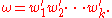Note that ω2 = 0 (i.e., ω is nilpotent of order 2), and moreover, for all . The following facts can be proven easily:
1. If n = 2k, then the left ideal Δ = Cℓ(V, g)ω is a minimal left ideal. Furthermore, this splits into the two spin spaces Δ+ = Cℓevenω and Δ- = Cℓoddω on restriction to the action of the even Clifford algebra.
2. If n = 2k+1, then the action of the unit vector u on the left ideal decomposes the space into a pair of isomorphic irreducible eigenspaces (both denoted by Δ), corresponding to the respective eigenvalues +1 and −1.

In detail, suppose for instance that n is even. Suppose that I is a non-zero left ideal contained in . We shall show that I must in fact be equal to by proving that it contains a nonzero scalar multiple of ω.

Fix a basis wi of W and a complementary basis wi′ of W′ so that
wiwj′ +wj′ wi = δij, and2 = 0, (wi′)2 = 0.

Note that any element of I must have the form αω, by virtue of our assumption that . Let αω ∈ I be any such element. Using the chosen basis, we may write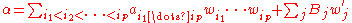where the ai1…ip are scalars, and the Bj are auxiliary elements of the Clifford algebra.
Observe now that the product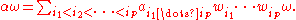Pick any nonzero monomial a in the expansion of α with maximal homogeneous degree in the elements wi: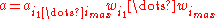(no summation implied),
thenis a nonzero scalar multiple of ω, as required.

Note that for n even, this computation also shows that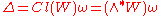.
as a vector space. In the last equality we again used that W is isotropic. In physics terms, this shows that Δ is built up like a Fock space
Fock space
The Fock space is an algebraic system used in quantum mechanics to describe quantum states with a variable or unknown number of particles. It is named after V. A...

by creating spinors using anti-commuting creation operators in W acting on a vacuum ω.

### Exterior algebra construction

The computations with the minimal ideal construction suggest that a spinor representation can
also be defined directly using the exterior algebra
Exterior algebra
In mathematics, the exterior product or wedge product of vectors is an algebraic construction used in Euclidean geometry to study areas, volumes, and their higher-dimensional analogs...

of the isotropic subspace W.
Let denote the exterior algebra of W considered as vector space only. This will be the spin representation, and its elements will be referred to as spinors.

The action of the Clifford algebra on Δ is defined first by giving the action of an element of V on Δ, and then showing that this action respects the Clifford relation and so extends to a homomorphism
Homomorphism
In abstract algebra, a homomorphism is a structure-preserving map between two algebraic structures . The word homomorphism comes from the Greek language: ὁμός meaning "same" and μορφή meaning "shape".- Definition :The definition of homomorphism depends on the type of algebraic structure under...

of the full Clifford algebra into the endomorphism ring
Endomorphism ring
In abstract algebra, one associates to certain objects a ring, the object's endomorphism ring, which encodes several internal properties of the object; this may be denoted End...

End(Δ) by the universal property of Clifford algebras. The details differ slightly according to whether the dimension of V is even or odd.

When dim(V) is even, where W′ is the chosen isotropic complement. Hence any decomposes uniquely as with and '. The action of v on a spinor is given by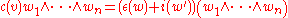where i(w′) is interior product with w′ using the non degenerate quadratic form to identify V with V*, and ε(w) denotes the exterior product. It is easily verified that
c(u)c(v) + c(v)c(u) = 2 g(u,v),

and so c respects the Clifford relations and extends to a homomorphism from the Clifford algebra to End(Δ).

The spin representation Δ further decomposes into a pair of irreducible complex representations of the Spin group (the half-spin representations, or Weyl spinors) via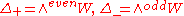.

When dim(V) is odd, , where U is spanned by a unit vector u orthogonal to W. The Clifford action c is defined as before on , while the Clifford action of (multiples of) u is defined by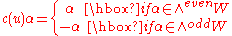As before, one verifies that c respects the Clifford relations, and so induces a homomorphism.

### Hermitian vector spaces and spinors

If the vector space V has extra structure which provides a decomposition of its complexification into two maximal isotropic subspaces, then the definition of spinors (by either method) becomes natural.

The main example is the case that the real vector space V is a hermitian vector space , i.e., V is equipped with a complex structure J which is an orthogonal transformation with respect to the inner product g on V. Then splits in the ±i eigenspaces of J. These eigenspaces are isotropic for the complexification of g and can be identified with the complex vector space and its complex conjugate . Therefore for a hermitian vector space the vector space ∧ (as well as its complex conjugate ∧V) is a spinor space for the underlying real euclidean vector space.

With the Clifford action as above but with contraction using the hermitian form, this construction gives a spinor space at every point of an almost Hermitian manifold and is the reason why every almost complex manifold
Almost complex manifold
In mathematics, an almost complex manifold is a smooth manifold equipped with smooth linear complex structure on each tangent space. The existence of this structure is a necessary, but not sufficient, condition for a manifold to be a complex manifold. That is, every complex manifold is an almost...

(in particular every symplectic manifold
Symplectic manifold
In mathematics, a symplectic manifold is a smooth manifold, M, equipped with a closed nondegenerate differential 2-form, ω, called the symplectic form. The study of symplectic manifolds is called symplectic geometry or symplectic topology...

) has a Spinc structure. Likewise, every complex vector bundle on a manifold carries a Spinc structure.

## Clebsch–Gordan decomposition

A number of Clebsch–Gordan decompositions are possible on the tensor product
Tensor product
In mathematics, the tensor product, denoted by ⊗, may be applied in different contexts to vectors, matrices, tensors, vector spaces, algebras, topological vector spaces, and modules, among many other structures or objects. In each case the significance of the symbol is the same: the most general...

of one spin representation with another. These decompositions express the tensor product in terms of the alternating representations of the orthogonal group.

For the real or complex case, the alternating representations are
• Γr = ∧rV, the representation of the orthogonal group on skew tensors of rank r.

In addition, for the real orthogonal groups, there are three characters
Character theory
In mathematics, more specifically in group theory, the character of a group representation is a function on the group which associates to each group element the trace of the corresponding matrix....

(one-dimensional representations)
• σ+ : O(p, q) → {−1, +1} given by σ+(R) = −1 if R reverses the spatial orientation of V, +1 if R preserves the spatial orientation of V. (The spatial character.)
• σ : O(p, q) → {−1, +1} given by σ(R) = −1 if R reverses the temporal orientation of V, +1 if R preserves the temporal orientation of V. (The temporal character.)
• σ = σ+σ . (The orientation character.)

The Clebsch–Gordan decomposition allows one to define, among other things:
• An action of spinors on vectors.
• A Hermitian metric on the complex representations of the real spin groups.
• A Dirac operator
Dirac operator
In mathematics and quantum mechanics, a Dirac operator is a differential operator that is a formal square root, or half-iterate, of a second-order operator such as a Laplacian...

on each spin representation.

### Even dimensions

If n = 2k is even, then the tensor product of Δ with the contragredient representation decomposes as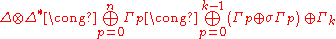which can be seen explicitly by considering (in the Explicit construction) the action of the Clifford algebra on decomposable elements αω ⊗ βω′. The rightmost formulation follows from the transformation properties of the Hodge star operator. Note that on restriction to the even Clifford algebra, the paired summands Γp ⊕ σΓp are isomorphic, but under the full Clifford algebra they are not.

There is a natural identification of Δ with its contragredient representation via the conjugation in the Clifford algebra: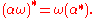So Δ ⊗ Δ also decomposes in the above manner. Furthermore, under the even Clifford algebra, the half-spin representations decomposeFor the complex representations of the real Clifford algebras, the associated reality structure on the complex Clifford algebra descends to the space of spinors (via the explicit construction in terms of minimal ideals, for instance). In this way, we obtain the complex conjugate of the representation Δ, and the following isomorphism is seen to hold: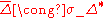In particular, note that the representation Δ of the orthochronous spin group is a unitary representation
Unitary representation
In mathematics, a unitary representation of a group G is a linear representation π of G on a complex Hilbert space V such that π is a unitary operator for every g ∈ G...

. In general, there are Clebsch–Gordan decompositions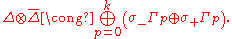In metric signature (p, q), the following isomorphisms hold for the conjugate half-spin representations
• If q is even, then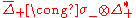and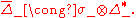• If q is odd, then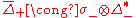andUsing these isomorphisms, one can deduce analogous decompositions for the tensor products of the half-spin representations .

### Odd dimensions

If n = 2k+1 is odd, then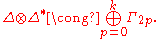In the real case, once again the isomorphism holds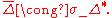Hence there is a Clebsch-Gordan decomposition (again using the Hodge star to dualize) given by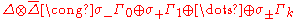### Consequences

There are many far-reaching consequences of the Clebsch–Gordan decompositions of the spinor spaces. The most fundamental of these pertain to Dirac's theory of the electron, among whose basic requirements are
• A manner of regarding the product of two spinors ψ as a scalar. In physical terms, a spinor should determine a probability amplitude
Probability amplitude
In quantum mechanics, a probability amplitude is a complex number whose modulus squared represents a probability or probability density.For example, if the probability amplitude of a quantum state is \alpha, the probability of measuring that state is |\alpha|^2...

for the quantum state.
• A manner of regarding the product ψ as a vector. This is an essential feature of Dirac's theory, which ties the spinor formalism to the geometry of physical space.
• A manner of regarding a spinor as acting upon a vector, by an expression such as ψv. In physical terms, this represents an electrical current of Maxwell's electromagnetic theory, or more generally a probability current
Probability current
In quantum mechanics, the probability current is a mathematical quantity describing the flow of probability density. Intuitively; if one pictures the probability density as an inhomogeneous fluid, then the probability current is the rate of flow of this fluid...

.

## Summary in low dimensions

• In 1 dimension (a trivial example), the single spinor representation is formally Majorana, a real
Real representation
In the mathematical field of representation theory a real representation is usually a representation on a real vector space U, but it can also mean a representation on a complex vector space V with an invariant real structure, i.e., an antilinear equivariant mapj\colon V\to V\,which...

1-dimensional representation that does not transform.
• In 2 Euclidean dimensions, the left-handed and the right-handed Weyl spinor are 1-component complex representation
Complex representation
The term complex representation has slightly different meanings in mathematics and physics.In mathematics, a complex representation is a group representationof a group on a complex vector space....

s, i.e. complex numbers that get multiplied by e±iφ/2 under a rotation by angle φ.
• In 3 Euclidean dimensions, the single spinor representation is 2-dimensional and quaternionic. The existence of spinors in 3 dimensions follows from the isomorphism of the group
Group (mathematics)
In mathematics, a group is an algebraic structure consisting of a set together with an operation that combines any two of its elements to form a third element. To qualify as a group, the set and the operation must satisfy a few conditions called group axioms, namely closure, associativity, identity...

s which allows us to define the action of Spin(3) on a complex 2-component column (a spinor); the generators of SU(2) can be written as Pauli matrices
Pauli matrices
The Pauli matrices are a set of three 2 × 2 complex matrices which are Hermitian and unitary. Usually indicated by the Greek letter "sigma" , they are occasionally denoted with a "tau" when used in connection with isospin symmetries...

.
• In 4 Euclidean dimensions, the corresponding isomorphism is . There are two inequivalent quaternionic 2-component Weyl spinors and each of them transforms under one of the SU(2) factors only.
• In 5 Euclidean dimensions, the relevant isomorphism is which implies that the single spinor representation is 4-dimensional and quaternionic.
• In 6 Euclidean dimensions, the isomorphism guarantees that there are two 4-dimensional complex Weyl representations that are complex conjugates of one another.
• In 7 Euclidean dimensions, the single spinor representation is 8-dimensional and real; no isomorphisms to a Lie algebra from another series (A or C) exist from this dimension on.
• In 8 Euclidean dimensions, there are two Weyl-Majorana real 8-dimensional representations that are related to the 8-dimensional real vector representation by a special property of Spin(8) called triality
Triality
In mathematics, triality is a relationship between three vector spaces, analogous to the duality relation between dual vector spaces. Most commonly, it describes those special features of the Dynkin diagram D4 and the associated Lie group Spin, the double cover of 8-dimensional rotation group SO,...

.
• In dimensions, the number of distinct irreducible spinor representations and their reality (whether they are real, pseudoreal, or complex) mimics the structure in d dimensions, but their dimensions are 16 times larger; this allows one to understand all remaining cases. See Bott periodicity.
• In spacetimes with p spatial and q time-like directions, the dimensions viewed as dimensions over the complex numbers coincide with the case of the -dimensional Euclidean space, but the reality projections mimic the structure in Euclidean dimensions. For example, in dimensions there are two non-equivalent Weyl complex (like in 2 dimensions) 2-component (like in 4 dimensions) spinors, which follows from the isomorphism .

Metric signature
Metric signature
The signature of a metric tensor is the number of positive and negative eigenvalues of the metric. That is, the corresponding real symmetric matrix is diagonalised, and the diagonal entries of each sign counted...

left-handed Weylright-handed WeylconjugacyDiracleft-handed Majorana-Weylright-handed Majorana-WeylMajorana
complex complex complex real real real
(2,0) 1 1 mutual 2
2
(1,1) 1 1 self 2 1 1 2
(3,0)
2
(2,1)
2
2
(4,0) 2 2 self 4
(3,1) 2 2 mutual 4
4
(5,0)
4
(4,1)
4
(6,0) 4 4 mutual 8
8
(5,1) 4 4 self 8
(7,0)
8
8
(6,1)
8
(8,0) 8 8 self 16 8 8 16
(7,1) 8 8 mutual 16
16
(9,0)
16
16
(8,1)
16
16

• Anyon
Anyon
In physics, an anyon is a type of particle that occurs only in two-dimensional systems. It is a generalization of the fermion and boson concept.-From theory to reality:...

• Dirac equation in the algebra of physical space
Dirac equation in the algebra of physical space
The Dirac equation, as the relativistic equation that describesspin 1/2 particles in quantum mechanics can be written in terms of the Algebra of physical space , which is a case of a Clifford algebra or geometric algebrathat is based in the use of paravectors....

• Plate trick
Plate trick
In mathematics and physics, the plate trick, also known as Feynman's plate trick, Dirac's belt trick, spinor spanner or quaternionic handshake is any of several particular physical demonstrations of the mathematical theorem that SU double-covers SO, or sometimes this fact itself...

• Pure spinor
Pure spinor
In a field of mathematics known as representation theory pure spinors are spinor representations of the special orthogonal group that are annihilated by the largest possible subspace of the Clifford algebra. They were introduced by Élie Cartan in the 1930s to classify complex structures...

• Spin-½
Spin-½
In quantum mechanics, spin is an intrinsic property of all elementary particles. Fermions, the particles that constitute ordinary matter, have half-integer spin. Spin-½ particles constitute an important subset of such fermions. All known elementary fermions have a spin of ½.- Overview :Particles...

• Spinor bundle
• Einstein–Cartan theory
Einstein–Cartan theory
In theoretical physics, the Einstein–Cartan theory, also known as the Einstein–Cartan–Sciama–Kibble theory or the Cartan–Sciama–Kibble theory is a classical theory of gravitation similar to general relativity but relaxing the assumption that the metric be torsion-free. Introducing torsion allows...

• Supercharge
Supercharge
In theoretical physics, a supercharge is a generator of supersymmetry transformations.Supercharge, denoted by the symbol Q, is an operator which transforms bosons into fermions, and vice versa...

• Twistor